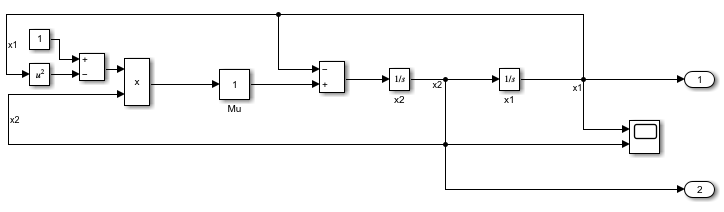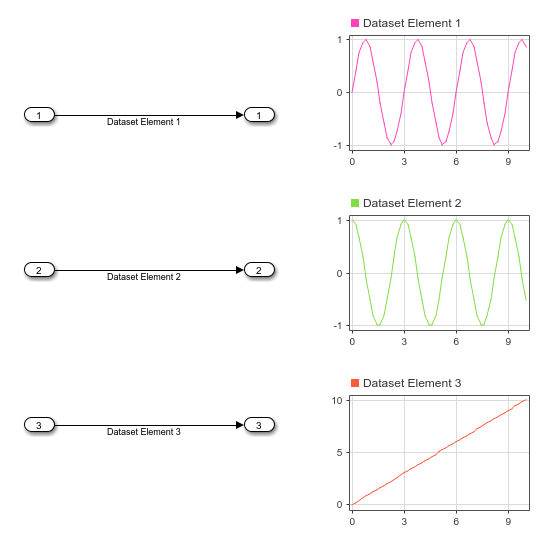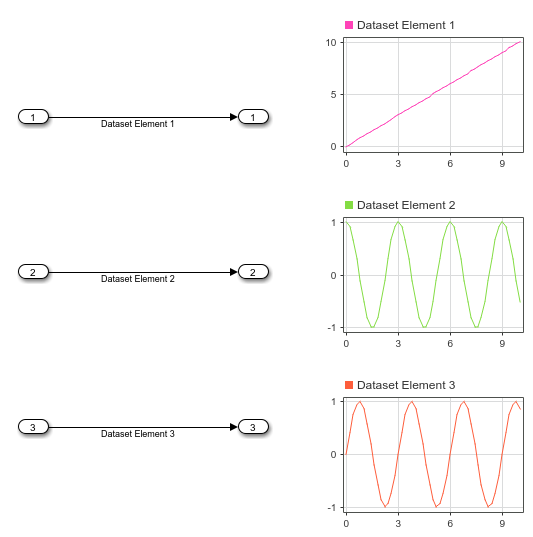Access logged simulation data or group simulation input data

## Description

A `Simulink.SimulationData.Dataset` object groups related data. Logged simulation results are often grouped in `Dataset` objects. You can also use a `Dataset` object to group simulation input data when you load initial states and when you load external input data using Inport blocks or the Signal Editor block.

A `Dataset` object created by logging simulation data contains one or more elements. Each element contains data for one logged signal, output, data store, or state. Each element is an object, and the type of the object depends on the data it contains.

When you create a `Dataset` object that groups simulation input data, each element contains data for a signal, bus, or array of buses. You can add data in any format supported by the loading method you use.

Type of InputData Formats

Scalar, vector, or multidimensional signal

Bus

• Structure of `timeseries`, `timetable`, or `matlab.io.datastore.SimulationDatastore` objects that matches the hierarchy of the bus

• `Simulink.SimulationData.Signal`

Array of buses

• Array of structures

• `Simulink.SimulationData.Signal`

Function-call signal

• N-by-1 vector

• `Simulink.SimulationData.Signal`

## Creation

Logging simulation data often creates a `Simulink.SimulationData.Dataset` object, including when you:

• Log data using signal logging.

• Log outputs, states, or final states using the `Dataset` format.

• Log data stores.

• Log data to the workspace or a MAT file using the Record block.

• Log data in `Dataset` format using a Scope block.

• Log data using a Floating Scope or Scope Viewer.

To group external input data for a model in a `Dataset` object, you can:

• Create an empty `Dataset` object and add the input data using the `addElement` function.

• Use the `createInputDataset` to create a `Dataset` object that contains an element for each root-level Inport block or In Bus Element block in a model. Then, specify the data for each element.

• Use the Signal Editor to interactively create and edit `Dataset` objects that contain simulation input data. For details, see Create and Edit Signal Data.

You can also create a `Dataset` object by converting data that uses another format to use the `Dataset` format. Having all data in a common format can facilitate postprocessing. For details, see Convert timeseries object to Dataset object.

### Syntax

``ds = Simulink.SimulationData.Dataset``
``ds = Simulink.SimulationData.Dataset(dataToConvert)``
``ds = Simulink.SimulationData.Dataset(dataToConvert,"DatasetName",dsName)``

### Description

example

````ds = Simulink.SimulationData.Dataset` creates an empty, unnamed `Dataset` object to which you can add elements. Use this syntax to manually create a `Dataset` object that contains external input data to load using Inport or In Bus Element blocks.```
````ds = Simulink.SimulationData.Dataset(dataToConvert)` creates an unnamed `Dataset` object that contains one or more elements that contain the data `dataToConvert`. Use this syntax to convert data that uses another format to use the `Dataset` format. For details, see Convert timeseries object to Dataset object.The conversion process only converts data for one input at a time. To convert data for multiple variables, convert each variable one at a time, then use the `concat` function to combine the resulting `Dataset` objects.```
````ds = Simulink.SimulationData.Dataset(dataToConvert,"DatasetName",dsName)` creates a `Dataset` object with the name specified by `dsName` that contains one or more elements that contain the data `dataToConvert`. Use this syntax to convert data that uses another format to use the `Dataset` format. For details, see Convert timeseries object to Dataset object.```

### Input Arguments

expand all

Data to convert to `Dataset` format, specified as a `timeseries` object, a structure, an array, or a `Simulink.ModelDataLogs` object. The results of conversion depend on the format of the input data.

Input DataConversion Results
`timeseries` object

`Dataset` object that contains one `Simulink.SimulationData.Signal` object with `timeseries` data in its `Values` property.

`ModelDataLogs` object

`Dataset` object that contains one or more `Simulink.SimulationData.Signal` objects that contain the data for each signal in the input object.

Structure that matches the `Structure with time` logging format.

`Dataset` object that contains one or more `Simulink.SimulationData.Signal` objects that contain the data for each signal in the input structure.

Structure that matches the `Structure` logging format

`Dataset` object that contains one or more `Simulink.SimulationData.Signal` objects that contain the data for each signal in the input structure.

Because the input does not contain time data, the conversion creates a time vector for each signal that starts at `0` and uses a sampling interval of `1`.

Array

`Dataset` object that contains one `Simulink.SimulationData.Signal` object. The `Values` property of the `Signal` object contains a `timeseries` object, and the `Data` property of the `timeseries` object contains the entire array.

Because the input does not contain time data, the conversion creates a time vector for the signal that starts at `0` uses a sampling interval of `1`.

For more information, see Convert timeseries object to Dataset object and Convert Data to Dataset Format.

`Dataset` object name, specified as a string or character vector.

### Output Arguments

expand all

`Dataset` object, returned as a `Simulink.SimulationData.Dataset` object. The `Dataset` object is empty when you do not specify input arguments. Create an empty `Dataset` object when you want to group simulation input data in a single variable.

When you specify input arguments, the `Dataset` object contains one or more elements that contain the input data. The results of conversion depend on the format of the input data.

Input DataConversion Results
`timeseries` object

`Dataset` object that contains one `Simulink.SimulationData.Signal` object with the `timeseries` data in its `Values` property.

`ModelDataLogs` object

`Dataset` object that contains one or more `Simulink.SimulationData.Signal` objects that contain the data for each signal in the input object.

Structure that matches the `Structure with time` logging format

`Dataset` object that contains one or more `Simulink.SimulationData.Signal` objects that contain the data for each signal in the input structure.

Structure that matches the `Structure` logging format

`Dataset` object that contains one or more `Simulink.SimulationData.Signal` objects that contain the data for each signal in the input structure.

Because the input does not contain time data, the conversion creates a time vector for each signal that starts at `0` and uses a sampling interval of `1`.

Array

`Dataset` object that contains one `Simulink.SimulationData.Signal` object. The `Values` property of the `Signal` object contains a `timeseries` object, and the `Data` property of the `timeseries` object contains the entire array.

Because the input does not contain time data, the conversion creates a time vector for the signal that starts at `0` uses a sampling interval of `1`.

For more information, see Convert timeseries object to Dataset object and Convert Data to Dataset Format.

## Properties

expand all

`Dataset` object name, specified as a string or character vector. When you create a `Dataset` object to group simulation input data, you specify the name for the `Dataset` object. The name you specify does not need to match the name of the variable that contains the `Dataset` object.

The `Name` property of `Dataset` objects created by logging simulation data is set to match the logging variable name specified in the model configuration parameters.

## Object Functions

 `addElement` Add element to end of `Simulink.SimulationData.Dataset` object `concat` Concatenate `Simulink.SimulationData.Dataset` object to another `Dataset` object `exportToPreviousRelease` Save a `Dataset` object to a MAT-file you can open in any release `extractTimetable` Extract data from `Simulink.SimulationData.Dataset` or `Simulink.SimulationData.Signal` objects into timetables `find` Get element or collection of elements from `Simulink.SimulationData.Dataset` object `get` Get element or collection of elements from `Simulink.SimulationData.Dataset` object `getElementNames` Return names of all elements in `Simulink.SimulationData.Dataset` object `numElements` Get number of elements in `Simulink.SimulationData.Dataset` object `plot` Plot data in `Simulink.SimulationData.Dataset` object in the Simulation Data Inspector `removeElement` Remove element from `Simulink.SimulationData.Dataset` object `setElement` Change `Simulink.SimulationData.Dataset` object element stored at specified index

## Examples

collapse all

Open the model `vdp`. The model produces two outputs `x1` and `x2`.

```mdl = "vdp"; open_system(mdl);```Simulate the model, logging block states along with the output data.

`out = sim(mdl,"SaveState","on");`

All logged data is returned in the single variable `out` as a `Simulink.SimulationOutput` object. The `SimulationOutput` object contains a `Simulink.SimulationData.Dataset` object that groups each kind of logged data.

`out`
```out = Simulink.SimulationOutput: tout: [64x1 double] xout: [1x1 Simulink.SimulationData.Dataset] yout: [1x1 Simulink.SimulationData.Dataset] SimulationMetadata: [1x1 Simulink.SimulationMetadata] ErrorMessage: [0x0 char] ```

Access the `Dataset` object `yout` that contains the logged output data using dot notation. The `Dataset` object contains a `Simulink.SimulationData.Signal` object for each output.

`outputs = out.yout`
```outputs = Simulink.SimulationData.Dataset 'yout' with 2 elements Name BlockPath ____ _________ 1 [1x1 Signal] x1 vdp/Out1 2 [1x1 Signal] x2 vdp/Out2 - Use braces { } to access, modify, or add elements using index. ```

The `Signal` object has metadata about the signal, including the path to the block and index of the port that produces the signal. Use the `getElement` function to access the `Signal` object that contains the data for signal `x1` by name. You can also use curly braces(`{}`) to access elements in a `Dataset` object by index.

`outputX1 = getElement(outputs,'x1')`
```outputX1 = Simulink.SimulationData.Signal Package: Simulink.SimulationData Properties: Name: 'x1' PropagatedName: '' BlockPath: [1x1 Simulink.SimulationData.BlockPath] PortType: 'inport' PortIndex: 1 Values: [1x1 timeseries] Methods, Superclasses ```

The signal data is stored in the `Values` property of the `Signal` object as a `timeseries` object.

`outputValsX1 = outputX1.Values`
``` timeseries Common Properties: Name: 'x1' Time: [64x1 double] TimeInfo: tsdata.timemetadata Data: [64x1 double] DataInfo: tsdata.datametadata ```

The time values are in the `Time` property of the `timeseries` object. The signal values are in the `Data` property.

`outputTimesX1 = outputValsX1.Time`
```outputTimesX1 = 64×1 0 0.0001 0.0006 0.0031 0.0157 0.0785 0.2844 0.5407 0.8788 1.2788 ⋮ ```
`outputDataX1 = outputValsX1.Data`
```outputDataX1 = 64×1 2.0000 2.0000 2.0000 2.0000 1.9998 1.9943 1.9379 1.8155 1.5990 1.2687 ⋮ ```

You can also access the time values or data values by combining the steps into a single line of code.

`outputDataX1 = getElement(out.yout,'x1').Values.Data`
```outputDataX1 = 64×1 2.0000 2.0000 2.0000 2.0000 1.9998 1.9943 1.9379 1.8155 1.5990 1.2687 ⋮ ```

Create data for three simulation input signals and group them in a `Dataset` object. A simple model loads the contents of the `Dataset` object using three root-level Inport blocks. Dashboard Scope blocks in the model display each signal created using the loaded input data.

First, create the signal data to load into the model. Use the expression in this example to create the evenly-spaced time vector for an input signal, especially when modeling discrete input signals. MATLAB® supports several other methods for creating an evenly-spaced vector, but other methods can introduce double-precision rounding errors in the time data, which can lead to unexpected simulation results.

```sampleTime = 0.01; numSteps = 1001; time = sampleTime*(0:numSteps-1); time = time';```

Create signal data for a sine signal, a cosine signal, and a linear signal.

```sineVals = sin(2*pi/3*time); cosVals = cos(2*pi/3*time); lineVals = time;```

Create a `timeseries` object to contain the data for each signal. Give each `timeseries` object a descriptive name so signals are easy to identify once they are grouped in the `Dataset` object.

```sineTS = timeseries(sineVals,time,'Name','Sine Wave'); cosTS = timeseries(cosVals,time,'Name','Cosine Wave'); lineTS = timeseries(lineVals,time,'Name','Line');```

Create a `Dataset` object and use the `addElement` function to add each `timeseries` object to the `Dataset` object.

```inputData = Simulink.SimulationData.Dataset; inputData.Name = 'inputData'; inputData = addElement(inputData,sineTS); inputData = addElement(inputData,cosTS); inputData = addElement(inputData,lineTS)```
```inputData = Simulink.SimulationData.Dataset 'inputData' with 3 elements Name BlockPath ___________ _________ 1 [1x1 timeseries] Sine Wave '' 2 [1x1 timeseries] Cosine Wave '' 3 [1x1 timeseries] Line '' - Use braces { } to access, modify, or add elements using index. ```

When you load external input data using root-level Inport blocks, you specify the data to load using the Input parameter in the Model Configuration Parameters on the Data Import/Export pane. Open the model `LoadInputDataset` and see that the Input parameter is specified as `inputData`.

`open_system('LoadInputDataset.slx');`

Simulate the model. The Dashboard Scope block connected to the first Inport block shows the sine signal, the Dashboard Scope block connected to the second Inport block shows the cosine signal, and the Dashboard Scope block connected to the third Inport block shows the linear signal.

`out = sim('LoadInputDataset.slx');`You can swap the order of elements in the `Dataset` object and see the change reflected in how the elements are mapped to the Inport blocks.

```inputData{1} = lineTS; inputData{3} = sineTS```
```inputData = Simulink.SimulationData.Dataset 'inputData' with 3 elements Name BlockPath ___________ _________ 1 [1x1 timeseries] Line '' 2 [1x1 timeseries] Cosine Wave '' 3 [1x1 timeseries] Sine Wave '' - Use braces { } to access, modify, or add elements using index. ```

Simulate the model again. The Dashboard Scope block that displays the first element now shows the line, and the Dashboard Scope block that displays the third element shows the sine wave, reflecting the new order of elements in the `Dataset` object.

`out = sim('LoadInputDataset.slx');`## Tips

• You can use curly braces (`{}`) to access, add, or modify an element in a `Dataset` object by index.

• To access, add, or modify an element of a `Dataset` object by name, use the `getElement`, `addElement`, and `setElement` functions.

• When you group simulation inputs using `Dataset` objects, you can use the Signal Editor or the Signal Editor block to easily change which `Dataset` object provides input for the simulation.

• When you load external input data from a `Dataset` object using root-level Inport blocks, you can use the Root Inport Mapper to map each element in the `Dataset` object to an Inport block by block name, block path, signal name, or port order. You can also write your own function to map the data. For more information, see Map Root Inport Signal Data.

• When you save data in a `Dataset` object to a MAT file, consider saving to a Version 7.3 MAT file if the contents of the `Dataset` object are too large to fit in memory. You can create a `Simulink.SimulationData.DatasetRef` that references a `Dataset` object in a MAT file without loading the data into memory for data processing or for loading big data. For more information, see Load Big Data for Simulations.

• To save a `Dataset` object to a MAT file that you can open in an earlier release, use the `exportToPreviousRelease` function instead of the `save` function.

• You can write your own reader to import data from a file into a `Dataset` object in the base workspace, the model workspace, or the Signal Editor using the `Simulink.io.FileType` class. For more information, see Create Custom File Type for Import to Signal Editor.

## Version History

Introduced in R2011a